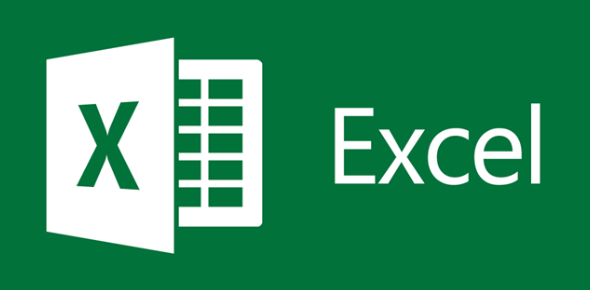# Basic MS Excel Trivia Exam: MCQ Quiz!

17 Questions | Total Attempts: 109SettingsWhat do you know about Basic MS Excel? Windows applications, such as Microsoft Access and Microsoft Word, and Excel, can communicate. Programmers have generated APIs to open Excel spreadsheets in a range of applications other than Microsoft Excel. For example, Excel spreadsheets can be retrieved from Python and can open Excel spreadsheets from Java Script. Basic MS Excel is ideal for learning. Don’t forget to attain your certificate after the quiz.

• 1.
If labels spill onto the column to its right & the column on the right is not empty you need to______
• A.

Re size the column

• B.

Complete your calculations by hand, excel is too hard to learn

• C.

Leave it the way it is

• D.

Delete and open new excel sheet

• 2.
A__________ is a single point of color on the screen.
• A.

Pixel

• B.

Cell

• C.

Border

• D.

Margin

• 3.
A value is always _____________ aligned in the cells & is included in calculations.
• A.

Left

• B.

Centered

• C.

Justified

• D.

Right

• 4.
This button allows you to incorporate the currency format to data.
• A.

.

• B.

%

• C.

=

• D.

-

• E.

\$

• 5.
When you key the date, excel applies a standard format that shows:
• A.

2 digits for the month, 2 digits for the day, & either 2 or 4 digits for the year.

• B.

Excel leaves it exactly the way I type it!

• C.

The formula to change the date.

• 6.
What does the function Sum do?
• A.

Adds the values in the range & then divides by the number of cells in the range.

• B.

Determines the largest value in a range.

• C.

Multiplies the values in a range.

• D.

Adds the values in the cell

• 7.
You can check results of your calculations by using:
• A.

Auto fit

• B.

Auto replace

• C.

Auto correct

• D.

Auto calculate

• 8.
A_________ is a built in mathematical formula included in excel.
• A.

Cell

• B.

Formula

• C.

Range

• D.

• E.

Function

• 9.
A________ is a series of instructions that performs a calculation & displays an answer.
• A.

Function

• B.

Formula

• C.

Range

• D.

Cell

• 10.
• A.

=

• B.

-

• C.

*

• D.

+

• 11.
A macro is a recording of a series of steps that completes a task or group of tasks that can be "played back" later when needed.
• A.

True

• B.

False

• 12.
When sorting, you must highlight the entire column of the column you wish to sort by.
• A.

True

• B.

False

• 13.
When creating a chart, you should never include a total of the data.
• A.

True

• B.

False

• 14.
To set up subtotals the data must first be formatted as a table.
• A.

True

• B.

False

• 15.
Conditional formatting allows you to restrict what data the user enters into a cell.
• A.

True

• B.

False

• 16.
How many data series can be used in a pie chart?
• A.

0

• B.

As many as you want

• C.

1

• D.

7

• 17.
You can use text in a formula.
• A.

True

• B.

False

Related TopicsBack to top